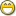### Re: How To Build Lookup Tables

euphoric said...
```sequence table = tables:new()

table = set(table,"a",123)
table = set(table,"b","Billy")
table = set(table,"c",{247,'x',{1,7,7}})
table = set(table,"x","Hawaii")
table = set(table,"y",77897)
table = set(table,"z",map:new())
```

Congratulations, you've created really slow maps!What are you looking for here, exactly? I know one problem we discussed previously was that save_map doesn't know how to store sub-maps. Are you trying to get around that?

-Greg